4.11.1 Destruction by Rotation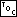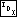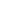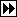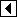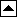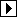To destroy a binary tree, we must visit and free each node. We have already covered one way to traverse a tree (inorder traversal) and used this technique for traversing and copying a binary tree. But, both times before, we were subject to both the explicit constraint that we had to visit the nodes in sorted order and the implicit constraint that we were not to change the structure of the tree, or at least not to change it for the worse.

Neither of these constraints holds for destruction of a binary tree. As long as the tree finally ends up freed, it doesn't matter how much it is mangled in the process. In this case, “the end justifies the means” and we are free to do it however we like.

So let's consider why we needed a stack before. It was to keep track of nodes whose left subtree we were currently visiting, in order to go back later and visit them and their right subtrees. Hmm...what if we rearranged nodes so that they didn't have any left subtrees? Then we could just descend to the right, without need to keep track of anything on a stack.

We can do this. For the case where the current node p has a left child q, consider the transformation below where we rotate right at p: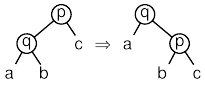where a, b, and c are arbitrary subtrees or even empty trees. This transformation shifts nodes from the left to the right side of the root (which is now q). If it is performed enough times, the root node will no longer have a left child. After the transformation, q becomes the current node.

For the case where the current node has no left child, we can just destroy the current node and descend to its right. Because the transformation used does not change the tree's ordering, we end up destroying nodes in inorder. It is instructive to verify this by simulating with paper and pencil the destruction of a few trees this way.

The code to implement destruction in this manner is brief and straightforward:

```84. <BST destruction function 84> =
void bst_destroy (struct bst_table *tree, bst_item_func *destroy) {
struct bst_node *p, *q;

assert (tree != NULL);

for (p = tree->bst_root; p != NULL; p = q)
if (p->bst_link == NULL)       {
if (destroy != NULL && p->bst_data != NULL)
destroy (p->bst_data, tree->bst_param);
tree->bst_alloc->libavl_free (tree->bst_alloc, p);
}     else       {